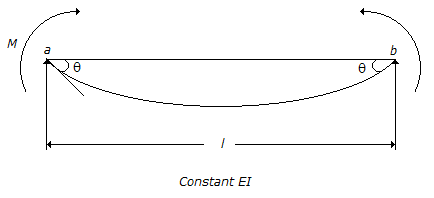# Civil Engineering - GATE Exam Questions

Exercise : GATE Exam Questions - Section 2
6.
The values of liquid limit and plasticity index for soils having common geological origin in a rectricted locality usually define
A zone above A-line
A straight line parallel to A-line
A straight line perpendicular to A-line
Points may be anywhere in the plasticity chart
Explanation:
No answer description is available. Let's discuss.

7.
M - θ relationship for a simply supported beam shown below is given by :M l/EI = 2θ
M l/EI = 3θ
M l/EI = 4θ
M l/EI = 6θ
Explanation:
No answer description is available. Let's discuss.

8.
The number of revolutions of a current meter in 50 seconds were found to be 12 and 30 corresponding to the velocities of 0.25 and 0.46 m/s respectively. What velocity (in m/s) would be indicated by 50 revolutions of that current meter in one minute ?
0.42
0.50
0.60
0.73
Explanation:
No answer description is available. Let's discuss.

9.
A lysimeter is used to measure :
infiltration
evaporation
evapotranspiration